# Inequalities and graphs example 4

Shade the region on the graph that would satisfy all the following inequalities, label this region R.

x<3       y>\-2       y<\x

Take each inequality separately

x<3

First let’s calculate points on the graph

y=-2       then      x is <3

y=-1       then      x is still <3

y=0             then      x is still <3

y=1             then      x is still <3

y=2             then      x is still <3

Now draw a graph and mark the above points.

Don’t forget to use a dotted line.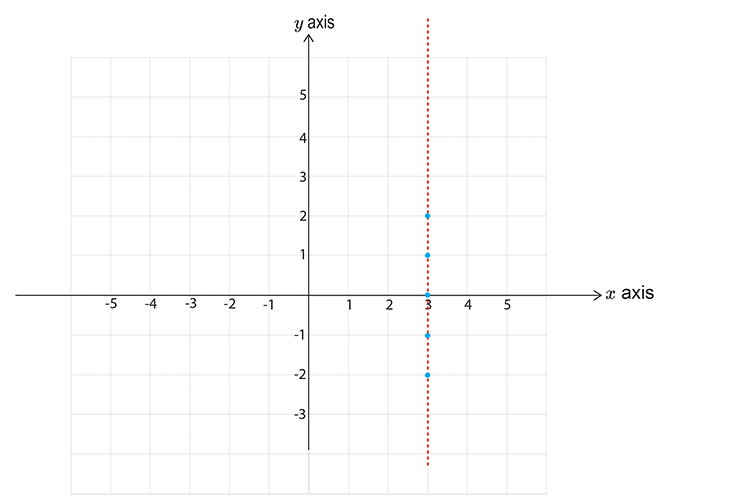Now pick a point NOT on the line.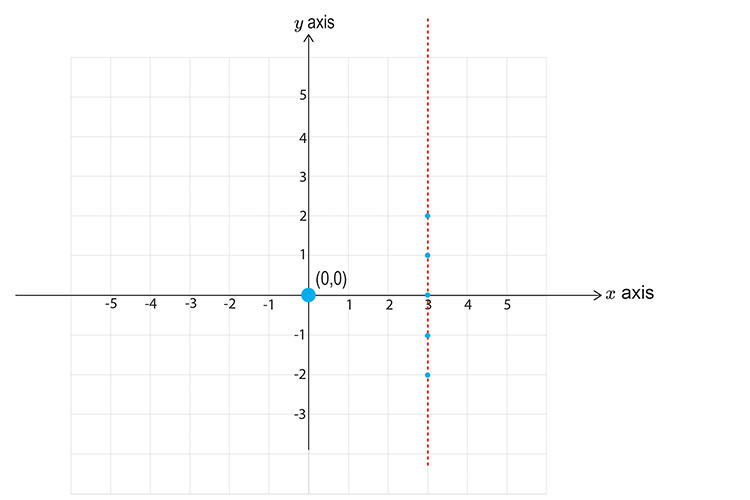Choose x=0   y=0

x<3

0<3

This reads zero is less than three.

This DOES satisfy the inequality, SHADE IT.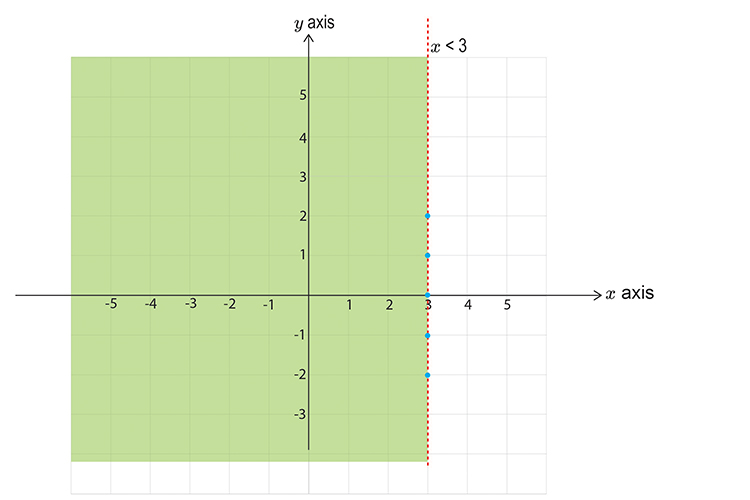Take the next inequality

y>\-2

First let’s calculate points on the graph

x=-2       then      y is >\-2

x=-1       then      y is >\-2

x=0             then      y is >\-2

x=1             then      y is >\-2

x=2             then      y is >\-2

Now draw a graph and mark the above points. Don’t forget to use a DOTTED LINE.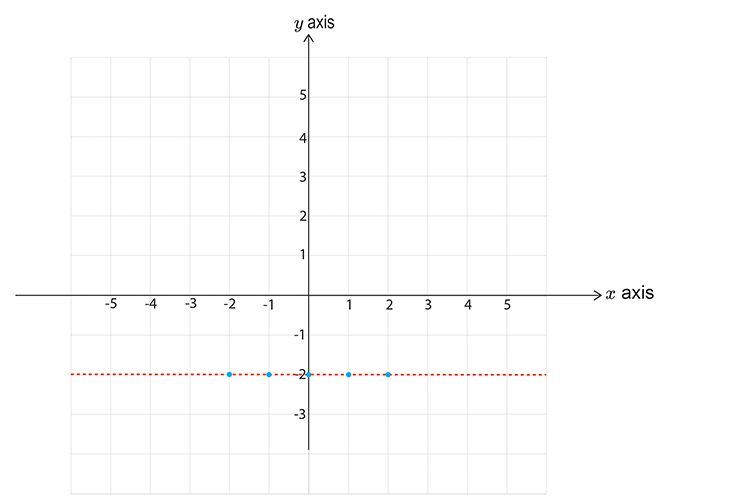Now pick a point NOT on the line.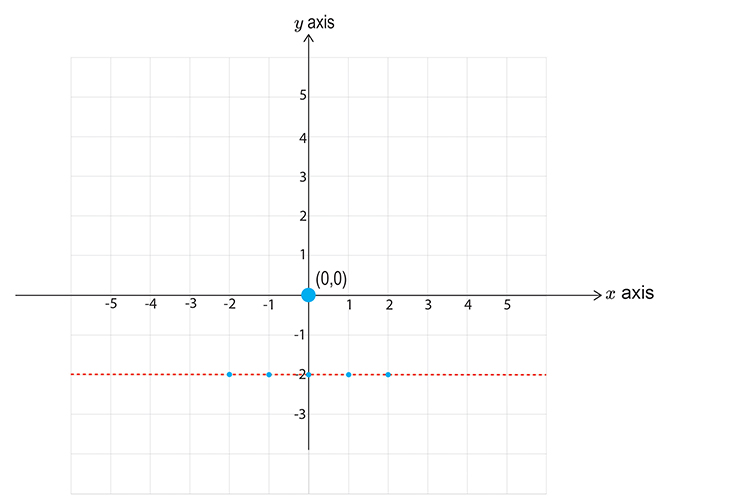Choose x=0     y=0

y>\-2

0>\-2

This reads zero is greater than minus two.

This DOES satisfy the inequality, SHADE IT.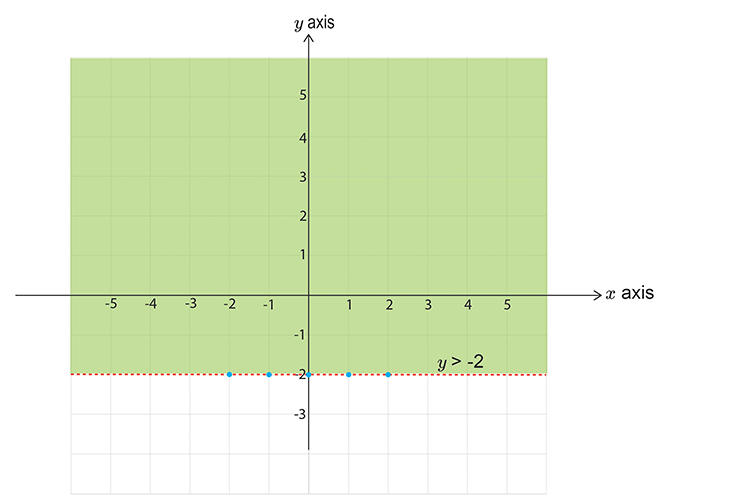Take the final inequality

y<x

First let’s calculate points on the graph

x=-2       then      y <-2

x=-1       then      y <-1

x=0             then      y <0

x=1             then      y <1

x=2             then      y <2

Now draw a graph and mark the above points. Don’t forget to draw a DOTTED line.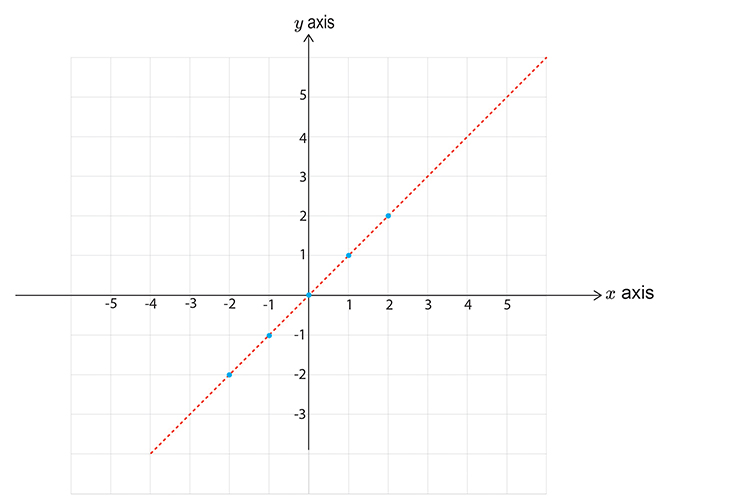Now pick a point NOT on the line.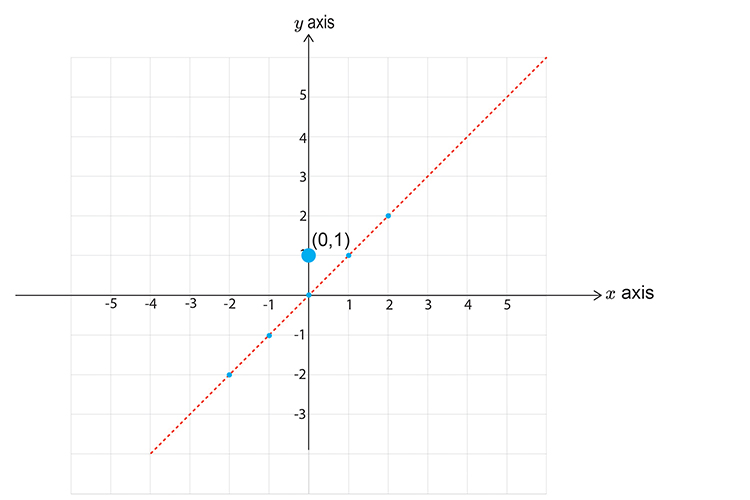Choose x=0       y=0

y<\x

1<\0

This reads one is less than zero, which is obviously wrong and therefore this does NOT satisfy the inequality, SHADE THE OTHER HALF.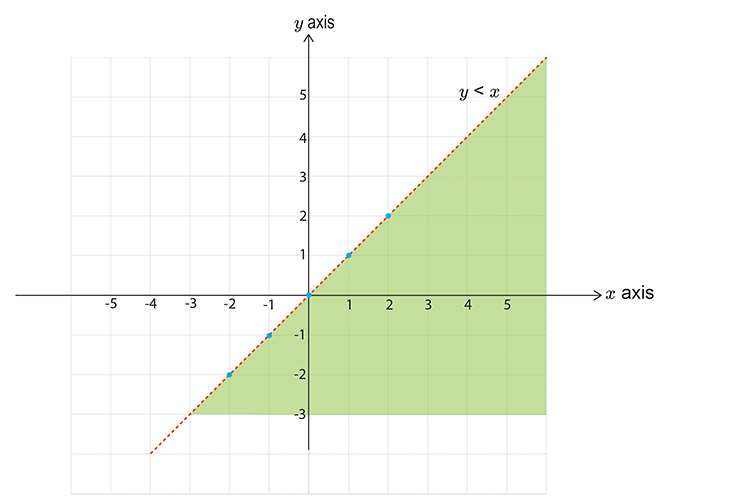Now plot all three graphs.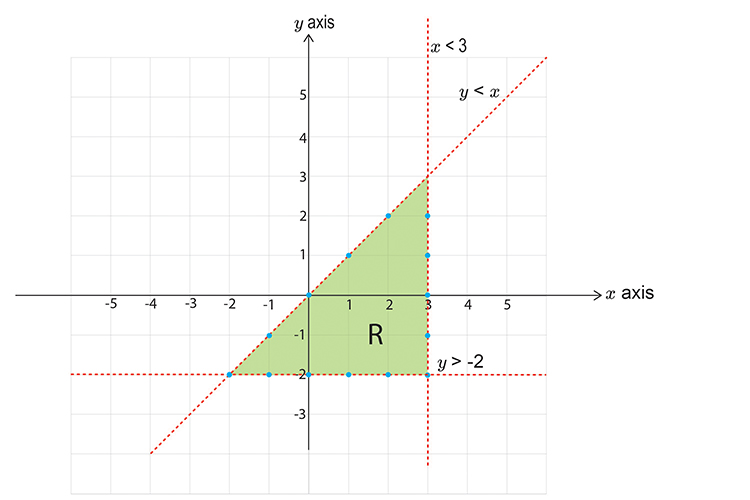R satisfies all the inequalities.